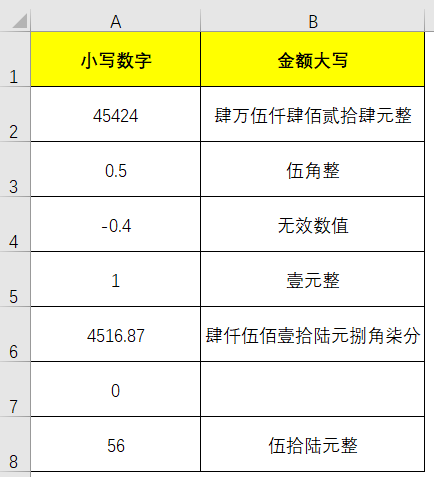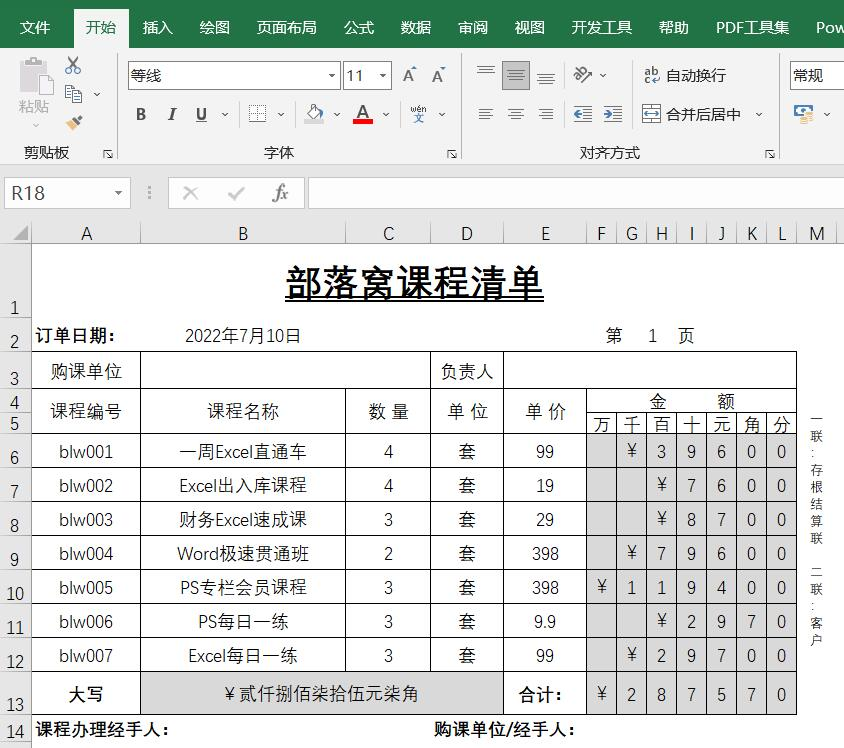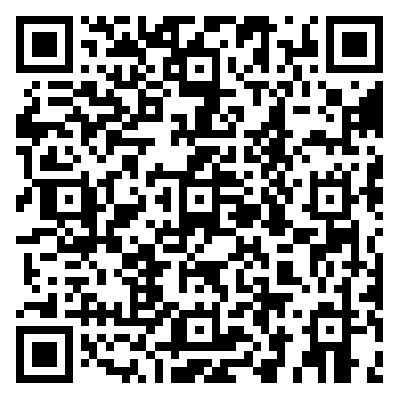# Excel表格小写数字转大写金额的公式分享以及票据模板赠送

[日期：2022-07-11]   来源：IT部落窝  作者：IT部落窝   阅读：0[字体： ]B2单元格输入excel小写数字转大写金额的公式为：
=IF(A2<0,"无效数值",IF(A2=0,"",IF(A2<1,"",TEXT(INT(A2),"[dbnum2]")&"元")&IF(INT(A2*10)-INT(A2)*10=0,IF(INT(A2)*(INT(A2*100)-INT(A2*10)*10)=0,"","零"),IF(AND((INT(A2)-INT(A2/10)*10)=0,INT(A2)>0),"零"&TEXT(INT(A2*10)-INT(A2)*10,"[dbnum2]")&"角",TEXT(INT(A2*10)-INT(A2)*10,"[dbnum2]")&"角"))&IF((INT(A2*100)-INT(A2*10)*10)=0,"整",TEXT(INT(A2*100)-INT(A2*10)*10,"[dbnum2]")&"分")))分享到： QQ空间 新浪微博 腾讯微博 人人网